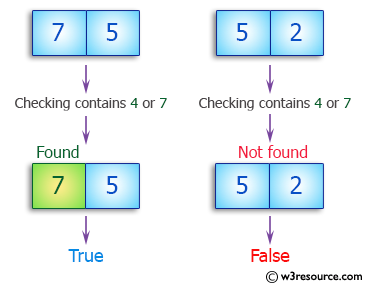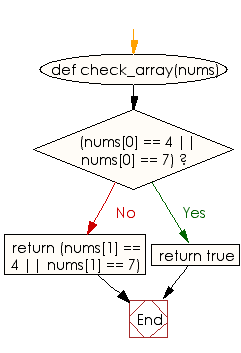﻿ Ruby Array exercises: Check whether a given array of integers of length 2 contains a 4 or a 7 - w3resource# Ruby Array Exercises: Check whether a given array of integers of length 2 contains a 4 or a 7

## Ruby Array: Exercise-17 with Solution

Write a Ruby program to check whether a given array of integers of length 2 contains a 4 or a 7.Ruby Code:

``````def check_array(nums)
if(nums == 4 || nums == 7)
return true
end
return (nums == 4 || nums == 7)
end

print check_array([1, 4]),"\n"
print check_array([7, 5]),"\n"
print check_array([5, 2])
``````

Output:

```true
true
false
```

Flowchart:Ruby Code Editor: